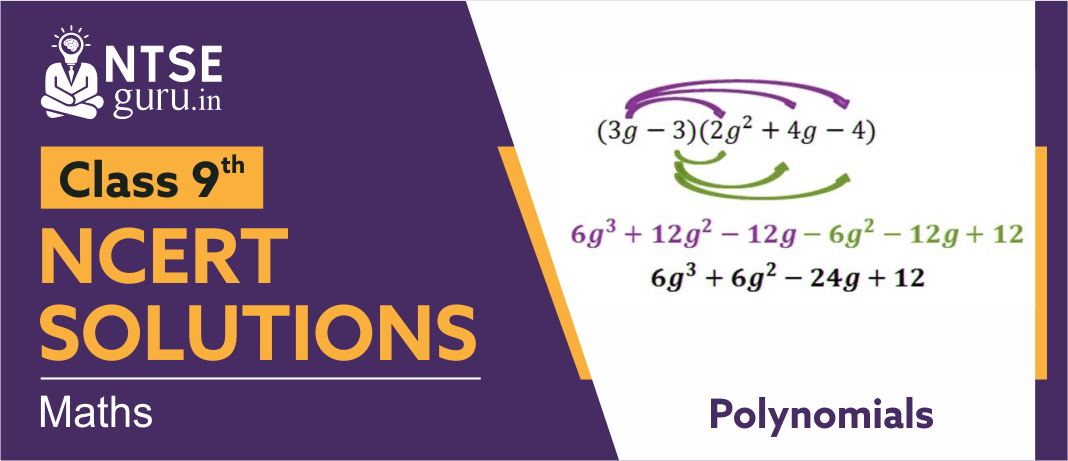# NCERT Solutions For Class 9 Maths Chapter 2NCERT  Solutions for Class 9 Maths Chapter 2, ‘Polynomials’ describes Remainder Theorem and Factor Theorem & their use in the factorisation of polynomials. In addition to the above, students will also study some important algebraic identities and their use in factorisation and evaluation of some given expressions

## Polynomials  -  Class 9 NCERT Solutions

‘Polynomials’ are referred to as the language of mathematics and algebra. They are used in nearly every field of mathematics to express numbers as a result of mathematical operations. To learn more about polynomials in depth, below are ‘Polynomials  -  Class 9 NCERT Solutions’ for your reference.

We hope the above solutions made it easy for you to consolidate the chapter.

For more solutions on the next chapters, you can follow us on www.ntseguru.in or can download our “ntse guru” app from the play store.